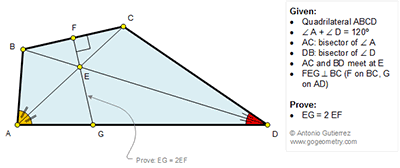## Saturday, January 16, 2016

### Geometry Problem 1180: Quadrilateral, 120 Degrees, Diagonals, Perpendicular

Geometry Problem. Post your solution in the comment box below.
Level: Mathematics Education, High School, Honors Geometry, College.

Click the figure below to view more details of problem 1180.1.Let AB, DC meet at H so that E is the incentre of Tr. AHD and < BGE = < DGE = 30.

Also easily < ACD = 60 + A/2 = < DBG ( A + 60 - A/2) making BECH concylic

Hence BE = CE = 2FE since Tr . ECF is 30-60-90.

Further Tr. s GED is congruent to Tr. CED case ASA making EG = EC

1.I am not sure from "E is the incentre of Tr. AHD" , you get " < BGE = < DGE = 30.

Peter

2.Just a typo replace < BGE = < DGE = 30 with < BHE = < DHE = 30

2.I could not complete the last line

Hence EG = 2FE

Sumith Peiris
Moratuwa
Sri Lanka

3.http://s1.postimg.org/kgkafchkf/pro_1180.png

BA meet CD at M
Since ∠(A)+ ∠(D)= 120 =>∠(M)=60
Since EA and ED bisect ∠(A) and ∠(D) => E is in center if trị. MAD and ME bisect ∠((M) and ∠( AED)=120
MBEC is cyclic and chord BE=chord EC => trị BED is isosceles
Triangle EFC is 30-60-90 triangle => EC= 2. EF
Triangles ECD and EDG are congruence…. ( case ASA) => EG=EC=2. EF

4.To Sumith & Peter
Why Tr CED congrc to EGD

5.ASA. ED common. <GDE = <CDE and < GED = < CED = 60 which I'm sure u can figure out why.

6.OK to Sumith
From congr in tr BGC, GF median and BM median (M on GC)
=>EG = 2FE

7.Interestingly Tr. BGC is equilateral from which the result EG = 2 FE is obvious.